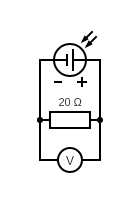# cell¶

Measure the voltage and current of a single solar panel cell.

## Hypothesis¶

The output of a single solar cell is around 2V and 200 mA based on a similar sized cell.

The solar cells were salvaged from some outdoor solar landscape lights that were replaced by hardwired ones. The voltage and current of the cells were unknown and so this test is meant to measure them. The cells are 57mm x 57mm and these ones on Amazon are 50mm x 50mm which is similar in size. The Amazon ones are 2V and 160mA and so I estimated that the 57mm ones are around 2V and 200 mA.

## Assumptions¶

• The multimeter is accurate.
• Ohm's Law is valid.
• The resistor can handle the power dissipation.
• The average solar radiation intensity at latitude 33.6020861 is 1000 W/m2.

## Procedure¶

### Circuit¶

Connect a 20Ω resistor to a solar cell.### Output¶

Measure the actual resistance of the resistor.

Measure the voltage across the resistor when the cell is in direct sun light.

$Resistance=20Ω$
$Voltage=2.212V$

## Analysis¶

### Current¶

The current through the resistor can be calculated using Ohm's law.

$I=\frac{V}{R}$

Using our measure values.

$I=\frac{2.212V}{20Ω}$
$\boxed{I=0.111A}$

### Power¶

The power through the resistor can be calculated using the following formula.

$P=IV$

Using our measure values.

$P=0.111A\times2.212V$
$\boxed{P=0.25W}$

### Efficiency¶

According to this site, the average solar radiation intensity is "1000 W/m2 on a clear day at solar noon in the summer months" at the Woods Hole Oceanographic Institution lattitude which is, 41.5255063.

$solar\;radiation\;intensity = 1000 \frac{W}{m^2}$

I know that there are a lot of factors that go into this value but this can be used as a very rough starting point.

$solar\;radiation\;intensity=\frac{0.25W}{(57mm)^2}\times\frac{(1000mm)^2}{1m^2}$
$\boxed{solar\;radiation\;intensity=77\frac{W}{m^2}}$
$efficiency=\frac{77\frac{W}{m^2}}{1000\frac{W}{m^2}}$
$\boxed{efficiency=7.7\%}$

## Conclusion¶

The overall voltage, current, and power of the cell was less than expecting when compared to the smaller one found on Amazon. Some reasons include the cell being cloudy and dirty, therefore not allowing the most light to get to the solar cells and the light intensity outside during testing was not the same as what the Amazon cells were tested at.

Overall, these cells should be sufficient and a good starting point to design the solar panel.

WIP

## Deconstruction¶

As stated above, the solare cells were salvaged from outdoor solar LED lights.

Remove the top of the light from the glass housing.

Remove the lid by unscrewing the philips screws.

Snip the wires to the solar cell.

Remove the caulking with a flathead screwdriver.

The top of the light with the solar cell.

Use a flathead screwdriver to pry underneath the cell to dislodge the adhesive. It's easiest if the top is rotated while holding the screwdriver.

The solar cell removed from the top.

Use a razor blade to remove the rest of the adhesive and caulking. A wire brush and a little water can also be used to remove the rest of the caulking.

WIP